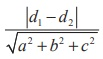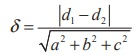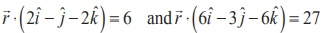Home | | Maths 12th Std | Distance between two parallel planes

# Distance between two parallel planes

Mathematics : The distance between two parallel planes

Distance between two parallel planes

### Theorem 6.21

The distance between two parallel planes ax + by + cz + d1 = 0 and ax + by + cz + d2 = 0 is given by### Proof

Let A( x1 , y1 , z1 ) be any point on the plane ax + by + cz + d2 = 0 , then we have

ax1 + by1 + cz1 + d2 = 0 ax1 + by1 + cz1 = −d2

The distance of the plane ax + by + cz + d1 = 0 from the point A( x1 , y1 , z1 ) is given byHence, the distance between two parallel planes ax + by + cz + d1 = 0 and ax + by + cz + d2 = 0 given by δ =.

### Example 6.51

Find the distance between the parallel planes x + 2 y − 2z +1 = 0 and 2x + 4 y − 4z + 5 = 0

Solution

We know that the formula for the distance between two parallel planes ax + by + cz + d1 = 0 and ax + by + cz + d2 = 0 isRewrite the second equation as x + 2y – 2z + 5/2 = 0. Comparing the given equations with the general equations, we get a = 1, b = 2, c = −2, d1=1, d2 = 5/2.

Substituting these values in the formula, we get the distanceExample 6.52

Find the distance between the planesSolution

Letbe the position vector of an arbitrary point on the plane(2 ˆi − ˆj − 2 ˆk ) = 6 . Then, we have(2 ˆ− ˆ− 2 ˆ) = 6                           .................(1)

If δ is the distance between the given planes, then δ is the perpendicular distance fromto the planeTags : Definition, Theorem, Proof, Solved Example Problems, Solution , 12th Mathematics : UNIT 6 : Applications of Vector Algebra
Study Material, Lecturing Notes, Assignment, Reference, Wiki description explanation, brief detail
12th Mathematics : UNIT 6 : Applications of Vector Algebra : Distance between two parallel planes | Definition, Theorem, Proof, Solved Example Problems, Solution